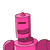# Complete the following flow chart to draw the tangents to the circle at points A and B.With centre P, draw a circle of radiu

Complete the following flow chart to draw the tangents to the circle at points A and B.
With centre P, draw a circle of radius 3 cm
and take any point A on it
Draw ray PA. Draw ray PB such that
ZAPB = 1000
Draw perpendicular lines to the ray PA and
ray PB at points A and B respectively.
Name the perpendicular lines as / and m.
I and in are tangents to the circle at
points A and B respectively.​

### 1 thought on “Complete the following flow chart to draw the tangents to the circle at points A and B.<br />With centre P, draw a circle of radiu”

1.